# WebGLFundamentals.org

Fix, Fork, Contribute

# WebGL 蒙皮

``````gl_Position = projection * view * model * position;
``````

``````gl_Position = projection * view *
(bone1Matrix * position * weight1 +
bone2Matrix * position * weight2 +
bone3Matrix * position * weight3 +
bone4Matrix * position * weight4);
``````

``````attribute vec4 a_position;
attribute vec4 a_weights;         // 每个顶点4个权重
attribute vec4 a_boneNdx;         // 4个骨骼下标
uniform mat4 bones[MAX_BONES];    // 每个骨骼1个矩阵

gl_Position = projection * view *
(bones[int(a_boneNdx)] * a_position * a_weight +
bones[int(a_boneNdx)] * a_position * a_weight +
bones[int(a_boneNdx)] * a_position * a_weight +
boneS[int(a_boneNdx)] * a_position * a_weight);
``````• `b0`, `b1``b2`是骨骼矩阵。
• `b1``b0`的子，`b2``b1`的子
• `0,1`对于骨骼b0的权重为1.0
• `2,3`对于骨骼b0和骨骼b1的权重都为0.5
• `4,5`对于骨骼b1的权重为1.0
• `6,7`对于骨骼b1和骨骼b2的权重都为0.5
• `8,9`对于骨骼b2的权重为1.0

``````var arrays = {
position: {
numComponents: 2,
data: [
0,  1,  // 0
0, -1,  // 1
2,  1,  // 2
2, -1,  // 3
4,  1,  // 4
4, -1,  // 5
6,  1,  // 6
6, -1,  // 7
8,  1,  // 8
8, -1,  // 9
],
},
boneNdx: {
numComponents: 4,
data: [
0, 0, 0, 0,  // 0
0, 0, 0, 0,  // 1
0, 1, 0, 0,  // 2
0, 1, 0, 0,  // 3
1, 0, 0, 0,  // 4
1, 0, 0, 0,  // 5
1, 2, 0, 0,  // 6
1, 2, 0, 0,  // 7
2, 0, 0, 0,  // 8
2, 0, 0, 0,  // 9
],
},
weight: {
numComponents: 4,
data: [
1, 0, 0, 0,  // 0
1, 0, 0, 0,  // 1
.5,.5, 0, 0,  // 2
.5,.5, 0, 0,  // 3
1, 0, 0, 0,  // 4
1, 0, 0, 0,  // 5
.5,.5, 0, 0,  // 6
.5,.5, 0, 0,  // 7
1, 0, 0, 0,  // 8
1, 0, 0, 0,  // 9
],
},

indices: {
numComponents: 2,
data: [
0, 1,
0, 2,
1, 3,
2, 3, //
2, 4,
3, 5,
4, 5,
4, 6,
5, 7, //
6, 7,
6, 8,
7, 9,
8, 9,
],
},
};
// 调用gl.createBuffer, gl.bindBuffer, gl.bufferData
var bufferInfo = webglUtils.createBufferInfoFromArrays(gl, arrays);
``````

``````// 4个矩阵, 每个骨骼一个
var numBones = 4;
var boneArray = new Float32Array(numBones * 16);

var uniforms = {
projection: m4.orthographic(-20, 20, -10, 10, -1, 1),
view: m4.translation(-6, 0, 0),
bones: boneArray,
color: [1, 0, 0, 1],
};
``````

``````// 为所有骨骼创建视图
// 在一个数组中以便上传，但是是分割的
// 数学计算用到的数组
var boneMatrices = [];  // 全局变量数据
var bones = [];         // 乘以绑定矩阵的逆之前的值
var bindPose = [];      // 绑定矩阵
for (var i = 0; i < numBones; ++i) {
boneMatrices.push(new Float32Array(boneArray.buffer, i * 4 * 16, 16));
bindPose.push(m4.identity());  // 仅仅分配存储空间
bones.push(m4.identity());     // 仅仅分配存储空间
}
``````

``````// 旋转每个骨骼角度，模拟一个层级
function computeBoneMatrices(bones, angle) {
var m = m4.identity();
m4.zRotate(m, angle, bones);
m4.translate(bones, 4, 0, 0, m);
m4.zRotate(m, angle, bones);
m4.translate(bones, 4, 0, 0, m);
m4.zRotate(m, angle, bones);
// bones没有用
}
``````

``````// 计算每个矩阵的初始位置
computeBoneMatrices(bindPose, 0);

// 计算他们的逆
var bindPoseInv = bindPose.map(function(m) {
return m4.inverse(m);
});
``````

``````var t = time * 0.001;
var angle = Math.sin(t) * 0.8;
computeBoneMatrices(bones, angle);
``````

``````// 每个都乘以绑定矩阵的逆
bones.forEach(function(bone, ndx) {
m4.multiply(bone, bindPoseInv[ndx], boneMatrices[ndx]);
});
``````

``````gl.useProgram(programInfo.program);
// 调用gl.bindBuffer, gl.enableVertexAttribArray, gl.vertexAttribPointer
webglUtils.setBuffersAndAttributes(gl, programInfo, bufferInfo);

// 调用gl.uniformXXX, gl.activeTexture, gl.bindTexture
webglUtils.setUniforms(programInfo, uniforms);

// 调用gl.drawArrays or gl.drawIndices
webglUtils.drawBufferInfo(gl, bufferInfo, gl.LINES);
````````````var ext = gl.getExtension('OES_texture_float');
if (!ext) {
return;  // 扩展在这个设备上不存在
}
``````

``````attribute vec4 a_position;
attribute vec4 a_weight;
attribute vec4 a_boneNdx;

uniform mat4 projection;
uniform mat4 view;
*uniform sampler2D boneMatrixTexture;
*uniform float numBones;

+// 这些偏移假设纹理每行4像素
+#define ROW0_U ((0.5 + 0.0) / 4.)
+#define ROW1_U ((0.5 + 1.0) / 4.)
+#define ROW2_U ((0.5 + 2.0) / 4.)
+#define ROW3_U ((0.5 + 3.0) / 4.)
+
+mat4 getBoneMatrix(float boneNdx) {
+  float v = (boneNdx + 0.5) / numBones;
+  return mat4(
+    texture2D(boneMatrixTexture, vec2(ROW0_U, v)),
+    texture2D(boneMatrixTexture, vec2(ROW1_U, v)),
+    texture2D(boneMatrixTexture, vec2(ROW2_U, v)),
+    texture2D(boneMatrixTexture, vec2(ROW3_U, v)));
+}

void main() {

gl_Position = projection * view *
*                (getBoneMatrix(a_boneNdx) * a_position * a_weight +
*                 getBoneMatrix(a_boneNdx) * a_position * a_weight +
*                 getBoneMatrix(a_boneNdx) * a_position * a_weight +
*                 getBoneMatrix(a_boneNdx) * a_position * a_weight);

}
```````````` (x + .5) / width
``````

`````` (0 + .5) / 3  = 0.166
(1 + .5) / 3 =  0.5
(2 + .5) / 3 =  0.833
````````````// 准备纹理来存骨骼矩阵
var boneMatrixTexture = gl.createTexture();
gl.bindTexture(gl.TEXTURE_2D, boneMatrixTexture);
// 因为我们希望使用纹理的原始数据
// 我们关闭筛选
gl.texParameteri(gl.TEXTURE_2D, gl.TEXTURE_MIN_FILTER, gl.NEAREST);
gl.texParameteri(gl.TEXTURE_2D, gl.TEXTURE_MAG_FILTER, gl.NEAREST);
// 也关闭包裹，因为纹理也许不是2的幂
gl.texParameteri(gl.TEXTURE_2D, gl.TEXTURE_WRAP_S, gl.CLAMP_TO_EDGE);
gl.texParameteri(gl.TEXTURE_2D, gl.TEXTURE_WRAP_T, gl.CLAMP_TO_EDGE);
``````

``````var uniforms = {
projection: m4.orthographic(-20, 20, -10, 10, -1, 1),
view: m4.translation(-6, 0, 0),
*  boneMatrixTexture,
*  numBones,
color: [1, 0, 0, 1],
};
``````

``````// 用此时的矩阵更新纹理
gl.bindTexture(gl.TEXTURE_2D, boneMatrixTexture);
gl.texImage2D(
gl.TEXTURE_2D,
0,         // 层级
gl.RGBA,   // 内部格式
4,         // 4像素宽,每个像素RGBA4个通道所以4像素是16个值
numBones,  // 每个骨骼一行
0,         // 边框
gl.RGBA,   // 格式
gl.FLOAT,  // 类型
boneArray);
``````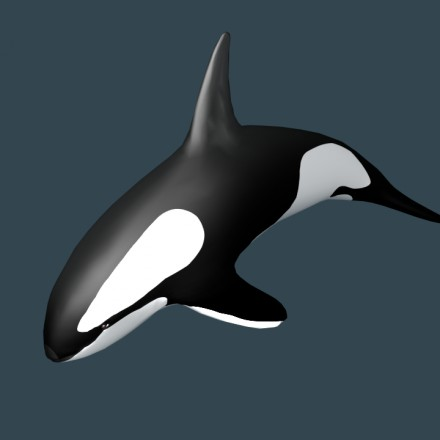glTF有两种格式。`.gltf`格式是一个JSON文件通常引用一个 `.bin`文件，这是一个二进制文件通常只包含几何体，可能包含动画数据。另一种格式是`.glb`二进制格式。通常是JSON和其他文件连接到一个二进制文件内，每个连接部分之间有一个短头和一个大小／类型描述。对于JavaScript，我认为`.gltf`格式稍微容易上手，所以让我们尝试加载它。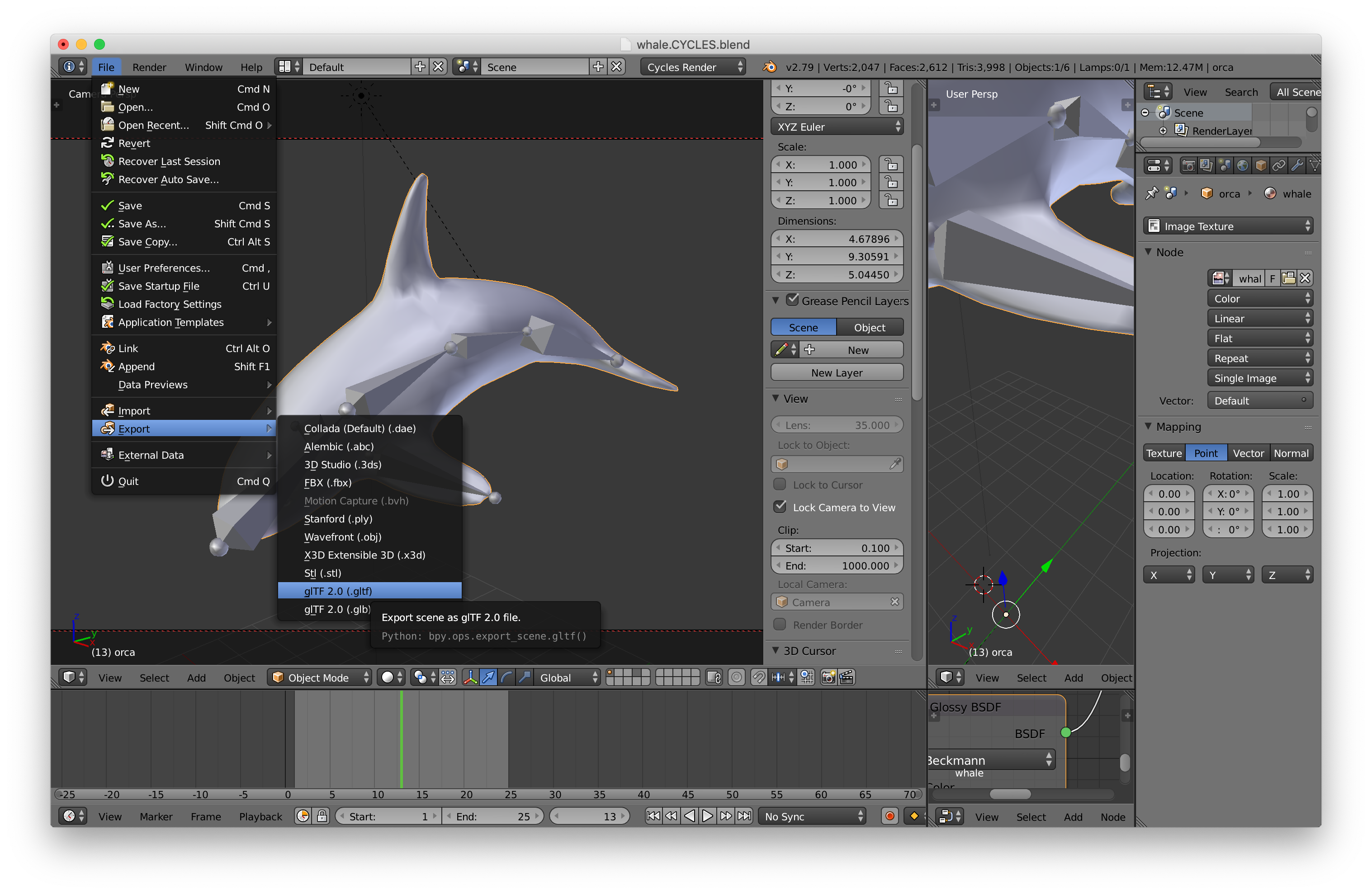``````async function loadGLTF(url) {

// 加载所有gltf文件相关连的文件
const baseURL = new URL(url, location.href);
gltf.buffers = await Promise.all(gltf.buffers.map((buffer) => {
const url = new URL(buffer.uri, baseURL.href);
}));

...

const response = await fetch(url);
if (!response.ok) {
throw new Error(`could not load: \${url}`);
}
return await response[typeFunc]();
}

}

}
``````

``````const cubeBufferInfo = {
attribs: {
'a_POSITION': { buffer: WebGLBuffer, type: gl.FLOAT, numComponents: 3, },
'a_NORMAL': { buffer: WebGLBuffer, type: gl.FLOAT, numComponents: 3, },
},
numElements: 24,
indices: WebGLBuffer,
elementType: gl.UNSIGNED_SHORT,
}
``````

``````// 给定一个访问器下标返回一个访问器, WebGLBuffer和一个stride
function getAccessorAndWebGLBuffer(gl, gltf, accessorIndex) {
const accessor = gltf.accessors[accessorIndex];
const bufferView = gltf.bufferViews[accessor.bufferView];
if (!bufferView.webglBuffer) {
const buffer = gl.createBuffer();
const target = bufferView.target || gl.ARRAY_BUFFER;
const arrayBuffer = gltf.buffers[bufferView.buffer];
const data = new Uint8Array(arrayBuffer, bufferView.byteOffset, bufferView.byteLength);
gl.bindBuffer(target, buffer);
gl.bufferData(target, data, gl.STATIC_DRAW);
bufferView.webglBuffer = buffer;
}
return {
accessor,
buffer: bufferView.webglBuffer,
stride: bufferView.stride || 0,
};
}
``````

``````function throwNoKey(key) {
throw new Error(`no key: \${key}`);
}

const accessorTypeToNumComponentsMap = {
'SCALAR': 1,
'VEC2': 2,
'VEC3': 3,
'VEC4': 4,
'MAT2': 4,
'MAT3': 9,
'MAT4': 16,
};

function accessorTypeToNumComponents(type) {
return accessorTypeToNumComponentsMap[type] || throwNoKey(type);
}
``````

``````const defaultMaterial = {
uniforms: {
u_diffuse: [.5, .8, 1, 1],
},
};

// 设置网格
gltf.meshes.forEach((mesh) => {
mesh.primitives.forEach((primitive) => {
const attribs = {};
let numElements;
for (const [attribName, index] of Object.entries(primitive.attributes)) {
const {accessor, buffer, stride} = getAccessorAndWebGLBuffer(gl, gltf, index);
numElements = accessor.count;
attribs[`a_\${attribName}`] = {
buffer,
type: accessor.componentType,
numComponents: accessorTypeToNumComponents(accessor.type),
stride,
offset: accessor.byteOffset | 0,
};
}

const bufferInfo = {
attribs,
numElements,
};

if (primitive.indices !== undefined) {
const {accessor, buffer} = getAccessorAndWebGLBuffer(gl, gltf, primitive.indices);
bufferInfo.numElements = accessor.count;
bufferInfo.indices = buffer;
bufferInfo.elementType = accessor.componentType;
}

primitive.bufferInfo = bufferInfo;

// 存储图元的材质信息
primitive.material = gltf.materials && gltf.materials[primitive.material] || defaultMaterial;
});
});
``````

``````class TRS {
constructor(position = [0, 0, 0], rotation = [0, 0, 0, 1], scale = [1, 1, 1]) {
this.position = position;
this.rotation = rotation;
this.scale = scale;
}
getMatrix(dst) {
dst = dst || new Float32Array(16);
m4.compose(this.position, this.rotation, this.scale, dst);
return dst;
}
}

class Node {
constructor(source, name) {
this.name = name;
this.source = source;
this.parent = null;
this.children = [];
this.localMatrix = m4.identity();
this.worldMatrix = m4.identity();
this.drawables = [];
}
setParent(parent) {
if (this.parent) {
this.parent._removeChild(this);
this.parent = null;
}
if (parent) {
this.parent = parent;
}
}
updateWorldMatrix(parentWorldMatrix) {
const source = this.source;
if (source) {
source.getMatrix(this.localMatrix);
}

if (parentWorldMatrix) {
// 一个矩阵传入，所以做数学运算
m4.multiply(parentWorldMatrix, this.localMatrix, this.worldMatrix);
} else {
// 没有矩阵传入，所以只是拷贝局部矩阵到世界矩阵
m4.copy(this.localMatrix, this.worldMatrix);
}

// 现在处理所有子
const worldMatrix = this.worldMatrix;
for (const child of this.children) {
child.updateWorldMatrix(worldMatrix);
}
}
traverse(fn) {
fn(this);
for (const child of this.children) {
child.traverse(fn);
}
}
this.children.push(child);
}
_removeChild(child) {
const ndx = this.children.indexOf(child);
this.children.splice(ndx, 1);
}
}
``````

• 此代码使用ES6的`class`特性。

使用`class`语法比定义类的旧方法要好得多。

• 我们给`Node`添加了要绘制的数组

这将列出从此节点要绘制的的物体。我们会用类的实例实际上来绘制。这个方法我们通常可以用不同的类绘制不同的物体。

注意：我不确定在Node里添加一个要绘制的数组是最好的方法。我觉得场景图本身应该可能不包含要绘制的物体。需要绘制的东西可改为图中节点的引用来获取数据。要绘制物体的方法比较常见所以让我们开始使用。

• 我们增加了一个`traverse`方法。

它用当前节点调用传入的函数，并对子节点递归执行。

• `TRS`类使用四元数进行旋转

我们并没有介绍过四元数，说实话我不认为我非常理解足以解释他们。幸运的是，我们用它们并不需要知道他们如何工作。我们只是从gltf文件中取出数据，调用一个函数它通过这些数据创建一个矩阵，使用该矩阵。

glTF文件中的节点数据存储为数组。 我们会转换glTF文件中的节点数据为`Node`实例。我们存储节点数据的旧数组为`origNodes`，我们稍后会需要用它。

``````const origNodes = gltf.nodes;
gltf.nodes = gltf.nodes.map((n) => {
const {name, skin, mesh, translation, rotation, scale} = n;
const trs = new TRS(translation, rotation, scale);
const node = new Node(trs, name);
const realMesh =　gltf.meshes[mesh];
if (realMesh) {
node.drawables.push(new MeshRenderer(realMesh));
}
return node;
});
``````

``````class MeshRenderer {
constructor(mesh) {
this.mesh = mesh;
}
render(node, projection, view, sharedUniforms) {
const {mesh} = this;
gl.useProgram(meshProgramInfo.program);
for (const primitive of mesh.primitives) {
webglUtils.setBuffersAndAttributes(gl, meshProgramInfo, primitive.bufferInfo);
webglUtils.setUniforms(meshProgramInfo, {
u_projection: projection,
u_view: view,
u_world: node.worldMatrix,
});
webglUtils.setUniforms(meshProgramInfo, primitive.material.uniforms);
webglUtils.setUniforms(meshProgramInfo, sharedUniforms);
webglUtils.drawBufferInfo(gl, primitive.bufferInfo);
}
}
}
``````

``````function addChildren(nodes, node, childIndices) {
childIndices.forEach((childNdx) => {
const child = nodes[childNdx];
child.setParent(node);
});
}

// 将节点加入场景图
gltf.nodes.forEach((node, ndx) => {
const children = origNodes[ndx].children;
if (children) {
}
});
``````

``````  // 设置场景
for (const scene of gltf.scenes) {
scene.root = new Node(new TRS(), scene.name);
}

return gltf;
}
``````

``````async function main() {
``````

``````const gltf = await loadGLTF('resources/models/killer_whale/whale.CYCLES.gltf');
``````

``````{
"name" : "orca",
"primitives" : [
{
"attributes" : {
"JOINTS_0" : 5,
"NORMAL" : 2,
"POSITION" : 1,
"TANGENT" : 3,
"TEXCOORD_0" : 4,
"WEIGHTS_0" : 6
},
"indices" : 0
}
]
}
``````

``````attribute vec4 a_POSITION;
attribute vec3 a_NORMAL;

uniform mat4 u_projection;
uniform mat4 u_view;
uniform mat4 u_world;

varying vec3 v_normal;

void main() {
gl_Position = u_projection * u_view * u_world * a_POSITION;
v_normal = mat3(u_world) * a_NORMAL;
}
``````

``````precision mediump float;

varying vec3 v_normal;

uniform vec4 u_diffuse;
uniform vec3 u_lightDirection;

void main () {
vec3 normal = normalize(v_normal);
float light = dot(u_lightDirection, normal) * .5 + .5;
gl_FragColor = vec4(u_diffuse.rgb * light, u_diffuse.a);
}
``````

``````// 编译和连接着色器，查找属性和全局变量的位置
const meshProgramInfo = webglUtils.createProgramInfo(gl, ["meshVS", "fs"]);
``````

``````const sharedUniforms = {
u_lightDirection: m4.normalize([-1, 3, 5]),
};

function renderDrawables(node) {
for(const drawable of node.drawables) {
drawable.render(node, projection, view, sharedUniforms);
}
}

for (const scene of gltf.scenes) {
// 更新场景中的世界矩阵。
scene.root.updateWorldMatrix();
// 遍历场景并渲染所有renderables
scene.root.traverse(renderDrawables);
}
``````

`renderDrawables`调用该节点上所有绘制对象的渲染方法，传入投影，视图矩阵，`sharedUniforms`包含的光照信息。

``````class Skin {
constructor(joints, inverseBindMatrixData) {
this.joints = joints;
this.inverseBindMatrices = [];
this.jointMatrices = [];
// 为每个关节矩阵分配足够的空间
this.jointData = new Float32Array(joints.length * 16);
// 为每个关节和绑定逆矩阵创建视图
for (let i = 0; i < joints.length; ++i) {
this.inverseBindMatrices.push(new Float32Array(
inverseBindMatrixData.buffer,
inverseBindMatrixData.byteOffset + Float32Array.BYTES_PER_ELEMENT * 16 * i,
16));
this.jointMatrices.push(new Float32Array(
this.jointData.buffer,
Float32Array.BYTES_PER_ELEMENT * 16 * i,
16));
}
// 创建存储关节矩阵的纹理
this.jointTexture = gl.createTexture();
gl.bindTexture(gl.TEXTURE_2D, this.jointTexture);
gl.texParameteri(gl.TEXTURE_2D, gl.TEXTURE_MIN_FILTER, gl.NEAREST);
gl.texParameteri(gl.TEXTURE_2D, gl.TEXTURE_MAG_FILTER, gl.NEAREST);
gl.texParameteri(gl.TEXTURE_2D, gl.TEXTURE_WRAP_S, gl.CLAMP_TO_EDGE);
gl.texParameteri(gl.TEXTURE_2D, gl.TEXTURE_WRAP_T, gl.CLAMP_TO_EDGE);
}
update(node) {
const globalWorldInverse = m4.inverse(node.worldMatrix);
// 遍历每个关节获得当前世界矩阵
// 来计算绑定矩阵的逆
// 并在纹理中存储整个结果
for (let j = 0; j < this.joints.length; ++j) {
const joint = this.joints[j];
const dst = this.jointMatrices[j];
m4.multiply(globalWorldInverse, joint.worldMatrix, dst);
m4.multiply(dst, this.inverseBindMatrices[j], dst);
}
gl.bindTexture(gl.TEXTURE_2D, this.jointTexture);
gl.texImage2D(gl.TEXTURE_2D, 0, gl.RGBA, 4, this.joints.length, 0,
gl.RGBA, gl.FLOAT, this.jointData);
}
}
``````

`MeshRenderer`一样，我们制作`SkinRenderer`，来用`Skin`来渲染蒙皮网格。

``````class SkinRenderer {
constructor(mesh, skin) {
this.mesh = mesh;
this.skin = skin;
}
render(node, projection, view, sharedUniforms) {
const {skin, mesh} = this;
skin.update(node);
gl.useProgram(skinProgramInfo.program);
for (const primitive of mesh.primitives) {
webglUtils.setBuffersAndAttributes(gl, skinProgramInfo, primitive.bufferInfo);
webglUtils.setUniforms(skinProgramInfo, {
u_projection: projection,
u_view: view,
u_world: node.worldMatrix,
u_jointTexture: skin.jointTexture,
u_numJoints: skin.joints.length,
});
webglUtils.setUniforms(skinProgramInfo, primitive.material.uniforms);
webglUtils.setUniforms(skinProgramInfo, sharedUniforms);
webglUtils.drawBufferInfo(gl, primitive.bufferInfo);
}
}
}
``````

``````<script id="skinVS" type="notjs">
attribute vec4 a_POSITION;
attribute vec3 a_NORMAL;
attribute vec4 a_WEIGHTS_0;
attribute vec4 a_JOINTS_0;

uniform mat4 u_projection;
uniform mat4 u_view;
uniform mat4 u_world;
uniform sampler2D u_jointTexture;
uniform float u_numJoints;

varying vec3 v_normal;

// 这些偏移假设纹理每行4个像素
#define ROW0_U ((0.5 + 0.0) / 4.)
#define ROW1_U ((0.5 + 1.0) / 4.)
#define ROW2_U ((0.5 + 2.0) / 4.)
#define ROW3_U ((0.5 + 3.0) / 4.)

mat4 getBoneMatrix(float jointNdx) {
float v = (jointNdx + 0.5) / u_numJoints;
return mat4(
texture2D(u_jointTexture, vec2(ROW0_U, v)),
texture2D(u_jointTexture, vec2(ROW1_U, v)),
texture2D(u_jointTexture, vec2(ROW2_U, v)),
texture2D(u_jointTexture, vec2(ROW3_U, v)));
}

void main() {
mat4 skinMatrix = getBoneMatrix(a_JOINTS_0) * a_WEIGHTS_0 +
getBoneMatrix(a_JOINTS_0) * a_WEIGHTS_0 +
getBoneMatrix(a_JOINTS_0) * a_WEIGHTS_0 +
getBoneMatrix(a_JOINTS_0) * a_WEIGHTS_0;
mat4 world = u_world * skinMatrix;
gl_Position = u_projection * u_view * world * a_POSITION;
v_normal = mat3(world) * a_NORMAL;
}
</script>
``````

``````*const globalWorldInverse = m4.inverse(node.worldMatrix);
// 遍历每个关节，获得它当前的世界矩阵
// 来计算绑定矩阵的逆
// 并在纹理中存储整个结果
for (let j = 0; j < this.joints.length; ++j) {
const joint = this.joints[j];
const dst = this.jointMatrices[j];
*  m4.multiply(globalWorldInverse, joint.worldMatrix, dst);
``````

``````+const skinNodes = [];
const origNodes = gltf.nodes;
gltf.nodes = gltf.nodes.map((n) => {
const {name, skin, mesh, translation, rotation, scale} = n;
const trs = new TRS(translation, rotation, scale);
const node = new Node(trs, name);
const realMesh =　gltf.meshes[mesh];
+  if (skin !== undefined) {
+    skinNodes.push({node, mesh: realMesh, skinNdx: skin});
+  } else if (realMesh) {
node.drawables.push(new MeshRenderer(realMesh));
}
return node;
});
``````

``````// 设置蒙皮
gltf.skins = gltf.skins.map((skin) => {
const joints = skin.joints.map(ndx => gltf.nodes[ndx]);
const {stride, array} = getAccessorTypedArrayAndStride(gl, gltf, skin.inverseBindMatrices);
return new Skin(joints, array);
});
``````

``````const glTypeToTypedArrayMap = {
'5120': Int8Array,    // gl.BYTE
'5121': Uint8Array,   // gl.UNSIGNED_BYTE
'5122': Int16Array,   // gl.SHORT
'5123': Uint16Array,  // gl.UNSIGNED_SHORT
'5124': Int32Array,   // gl.INT
'5125': Uint32Array,  // gl.UNSIGNED_INT
'5126': Float32Array, // gl.FLOAT
}

// 给定一个GL类型返回需要的类型
function glTypeToTypedArray(type) {
return glTypeToTypedArrayMap[type] || throwNoKey(type);
}

// 给定一个访问器下标返回访问器
// 和缓冲正确部分的类型化数组
function getAccessorTypedArrayAndStride(gl, gltf, accessorIndex) {
const accessor = gltf.accessors[accessorIndex];
const bufferView = gltf.bufferViews[accessor.bufferView];
const TypedArray = glTypeToTypedArray(accessor.componentType);
const buffer = gltf.buffers[bufferView.buffer];
return {
accessor,
array: new TypedArray(
buffer,
bufferView.byteOffset + (accessor.byteOffset || 0),
accessor.count * accessorTypeToNumComponents(accessor.type)),
stride: bufferView.byteStride || 0,
};
}
``````

``````// 给蒙皮节点添加SkinRenderers
for (const {node, mesh, skinNdx} of skinNodes) {
node.drawables.push(new SkinRenderer(mesh, gltf.skins[skinNdx]));
}
``````

``````const origMatrix = new Map();
function animSkin(skin, a) {
for(let i = 0; i < skin.joints.length; ++i) {
const joint = skin.joints[i];
// 如果这个关节并没有存储矩阵
if (!origMatrix.has(joint)) {
// 为关节存储一个矩阵
origMatrix.set(joint, joint.source.getMatrix());
}
// 获取原始的矩阵
const origMatrix = origRotations.get(joint);
// 旋转它
const m = m4.xRotate(origMatrix, a);
// 分解回关节的位置，旋转量，缩放量
m4.decompose(m, joint.source.position, joint.source.rotation, joint.source.scale);
}
}
``````

``````animSkin(gltf.skins, Math.sin(time) * .5);
``````

``````  gl_Position = u_projection * u_view *  a_POSITION;
``````

``````gl_FragColor = vec4(1, 0, 0, 1);
``````

``````const cameraPosition = [5, 0, 5];
const target = [0, 0, 0];
``````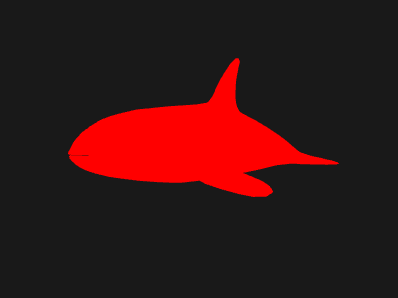``````gl_FragColor = vec4(normalize(v_normal) * .5 + .5, 1);
``````

``````v_normal = a_NORMAL;
``````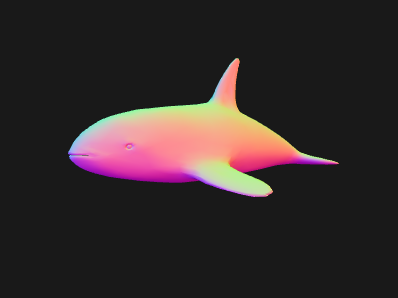``````v_normal = a_WEIGHTS_0.xyz * 2. - 1.;
``````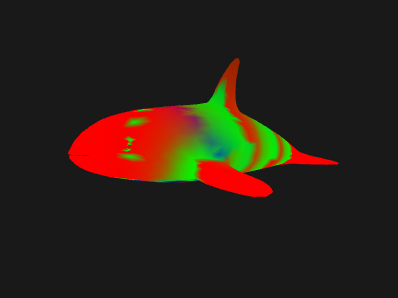``````v_normal = a_JOINTS_0.xyz / (u_numJoints - 1.) * 2. - 1.;
``````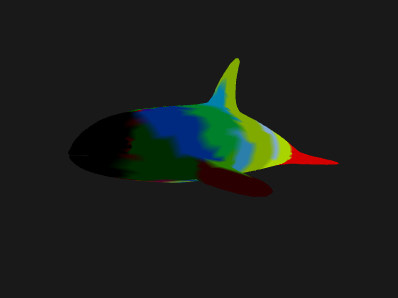• 基础概念
• 图像处理
• 二维平移，旋转，缩放和矩阵运算
• 三维
• 光照
• 组织和重构
• 几何
• 纹理
• 渲染到纹理
• 阴影
• 技术
• 建议
• 优化
• 杂项
• 参考

Issue/Bug? 在GitHub上提issue.